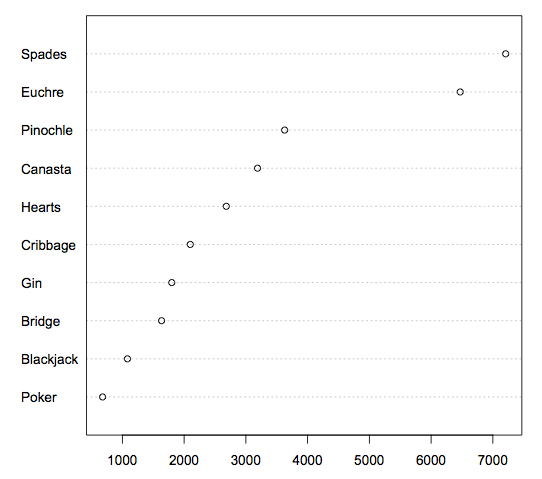# 2.10: Dot Plots

•• David Lane
• Rice University
$$\newcommand{\vecs}{\overset { \rightharpoonup} {\mathbf{#1}} }$$ $$\newcommand{\vecd}{\overset{-\!-\!\rightharpoonup}{\vphantom{a}\smash {#1}}}$$$$\newcommand{\id}{\mathrm{id}}$$ $$\newcommand{\Span}{\mathrm{span}}$$ $$\newcommand{\kernel}{\mathrm{null}\,}$$ $$\newcommand{\range}{\mathrm{range}\,}$$ $$\newcommand{\RealPart}{\mathrm{Re}}$$ $$\newcommand{\ImaginaryPart}{\mathrm{Im}}$$ $$\newcommand{\Argument}{\mathrm{Arg}}$$ $$\newcommand{\norm}{\| #1 \|}$$ $$\newcommand{\inner}{\langle #1, #2 \rangle}$$ $$\newcommand{\Span}{\mathrm{span}}$$ $$\newcommand{\id}{\mathrm{id}}$$ $$\newcommand{\Span}{\mathrm{span}}$$ $$\newcommand{\kernel}{\mathrm{null}\,}$$ $$\newcommand{\range}{\mathrm{range}\,}$$ $$\newcommand{\RealPart}{\mathrm{Re}}$$ $$\newcommand{\ImaginaryPart}{\mathrm{Im}}$$ $$\newcommand{\Argument}{\mathrm{Arg}}$$ $$\newcommand{\norm}{\| #1 \|}$$ $$\newcommand{\inner}{\langle #1, #2 \rangle}$$ $$\newcommand{\Span}{\mathrm{span}}$$

Learning Objectives

• Create and interpret dot plots
• Judge whether a dot plot would be appropriate for a given data set

Dot plots can be used to display various types of information. Figure $$\PageIndex{1}$$ uses a dot plot to display the number of M & M's of each color found in a bag of M & M's. Each dot represents a single M & M. From the figure, you can see that there were $$3$$ blue M & M's, $$19$$ brown M & M's, etc.Figure $$\PageIndex{1}$$: A dot plot showing the number of M & M's of various colors in a bag of M & M's

The dot plot in Figure $$\PageIndex{2}$$ shows the number of people playing various card games on the Yahoo website on a Wednesday. Unlike Figure $$\PageIndex{1}$$, the location rather than the number of dots represents the frequency.Figure $$\PageIndex{2}$$: A dot plot showing the number of people playing various card games on a Wednesday

The dot plot in Figure $$\PageIndex{3}$$ shows the number of people playing on a Sunday and on a Wednesday. This graph makes it easy to compare the popularity of the games separately for the two days, but does not make it easy to compare the popularity of a given game on the two days.Figure $$\PageIndex{3}$$: A dot plot showing the number of people playing various card games on a Sunday and on a WednesdayFigure $$\PageIndex{4}$$: An alternate way of showing the number of people playing various card games on a Sunday and on a Wednesday

The dot plot in Figure $$\PageIndex{4}$$ makes it easy to compare the days of the week for specific games while still portraying differences among games.

This page titled 2.10: Dot Plots is shared under a Public Domain license and was authored, remixed, and/or curated by David Lane via source content that was edited to the style and standards of the LibreTexts platform; a detailed edit history is available upon request.